# Low Dimensional Examples

#### 2023-05-03

library(hmer)
library(lhs)
library(ggplot2)
#> RStudio Community is a great place to get help: https://community.rstudio.com/c/tidyverse
set.seed(1)

# One-dimensional Example: Sine Function

## Setup

We first look at the simplest possible example: a single univariate function. We take the following sine function:

$$f(x) = 2x + 3x\sin\left(\frac{5\pi(x-0.1)}{0.4}\right).$$

This should demonstrate the main features of emulation and history matching over a couple of waves. The other advantage of using such a simple function (in this case as well as in the later, two-dimensional, case) is that the function can be evaluated quickly, so we can compare the emulator performance against the actual function value very easily. This is seldom the case in real-world applications, where a simulator is often a ‘black box’ function that can take a long time to evaluate at any given point.

func <- function(x) {
2*x + 3*x*sin(5*pi*(x-0.1)/0.4)
}

We presume that we want to emulate this function over the input space $$x\in[0,0.6]$$. To train an emulator to this function, we require a set of known points. We’ll evaluate the function at equally spaced points along the range $$[0, 0.5]$$: ten points will be sufficient for training this one-dimensional emulator. A general rule of thumb is that we require a number of points equal to at least ten times the dimension of the input space.

data1d <- data.frame(x = seq(0.05, 0.5, by = 0.05), f = func(seq(0.05, 0.5, by = 0.05)))

These points will be passed to the hmer package functions, in order for it to train an emulator to func, interpolate points between the data points above, and propose a set of new points for training a second-wave emulator.

## Emulator Training

To train the emulator, we require at least three things: the data to train on, the names of the outputs to emulate, and the parameter ranges. The function emulator_from_data can then be used to determine prior specifications for the emulator; namely expectations and variances of its component parts, as well as correlation lengths and other structural elements. It then performs Bayes Linear adjustment on this prior emulator to give us our trained emulator. To see these elements in more detail, consult the section “The Structure of a Bayes Linear Emulator” at the bottom of this document.

We therefore define the ranges of the parameters (in this case, just one parameter $$x$$) and use the emulator_from_data function, producing objects of class Emulator:

ranges1d <- list(x = c(0, 0.6))
em1d <- emulator_from_data(data1d, c('f'), ranges1d)
#> Fitting regression surfaces...
#> Building correlation structures...
#> Creating emulators...
em1d
#> $f #> Parameters and ranges: x: c(0, 0.6) #> Specifications: #> Basis functions: (Intercept) #> Active variables x #> Regression Surface Expectation: 0.5963 #> Regression surface Variance (eigenvalues): 0 #> Correlation Structure: #> Bayes-adjusted emulator - prior specifications listed. #> Variance (Representative): 7.008676 #> Expectation: 0 #> Correlation type: exp_sq #> Hyperparameters: theta: 0.3333 #> Nugget term: 0 #> Mixed covariance: 0 The print statement for the emulator shows us the specifications: the basis functions chosen, the corresponding regression coefficients, the global variance, and the structure and hyperparameters of the correlation structure. We also note that the output of emulator_from_data is a named list of emulators: here, this is not particularly important as we only have one emulator. The print statement also indicates that Bayes Linear adjustment has been applied: if we instead wanted to examine an unadjusted emulator, we could have run emulator_from_data with the option adjusted = FALSE, or having trained an emulator we can access the prior emulator by calling o_em. The below commands give the same output. emulator_from_data(data1d, c('f'), ranges1d, adjusted = FALSE)$f
#> Fitting regression surfaces...
#> Building correlation structures...
#> Creating emulators...
#> Parameters and ranges:  x: c(0, 0.6)
#> Specifications:
#>   Basis functions:  (Intercept)
#>   Active variables x
#>   Regression Surface Expectation:  0.5963
#>   Regression surface Variance (eigenvalues):  0
#> Correlation Structure:
#>   Variance (Representative):  7.008676
#>   Expectation:  0
#>   Correlation type: exp_sq
#>   Hyperparameters:  theta: 0.3333
#>   Nugget term: 0
#> Mixed covariance:  0
em1d$f$o_em
#> Parameters and ranges:  x: c(0, 0.6)
#> Specifications:
#>   Basis functions:  (Intercept)
#>   Active variables x
#>   Regression Surface Expectation:  0.5963
#>   Regression surface Variance (eigenvalues):  0
#> Correlation Structure:
#>   Variance (Representative):  7.008676
#>   Expectation:  0
#>   Correlation type: exp_sq
#>   Hyperparameters:  theta: 0.3333
#>   Nugget term: 0
#> Mixed covariance:  0

The trained emulator, by virtue of having been provided the training points, ‘knows’ the value of the function at those points. Hence, the expectation of the emulator at those points is identical (up to numerical precision) to the known values of $$f(x)$$, and the variance at those points is $$0$$. We can access this information using the built-in functions get_exp and get_cov of the Emulator object.

em1d$f$get_exp(data1d) - data1d$f #>  9.489274e-10 -6.827872e-15 -2.142730e-14 -4.418688e-14 -5.717649e-14 -3.508305e-14 2.249818e-10 #>  9.992007e-16 9.325873e-15 -2.220446e-16 em1d$f$get_cov(data1d) #> 1 2 3 4 5 6 7 8 9 10 #> 0 0 0 0 0 0 0 0 0 0 We can now use this trained emulator to evaluate the function at any point in the parameter range. While it will not exactly match the function value, each evaluation comes with its associated uncertainty. We define a ‘large’ set of additional points to evaluate the emulator on, and find their expectation and variance. test1d <- data.frame(x = seq(0, 0.6, by = 0.001)) em1d_exp <- em1d$f$get_exp(test1d) em1d_var <- em1d$f$get_cov(test1d) Since, by design, we have a function that is quick and easy to evaluate, we can directly compare the emulator predictions against the true function value, plotting the relevant quantities. In higher-dimensional cases, the hmer package has built-in plotting functionality, but here we use simple R plotting methods. #> Define a data.frame for the plotting plot1d <- data.frame( x = test1d$x,
f = func(test1d$x), E = em1d_exp, max = em1d_exp + 3*sqrt(abs(em1d_var)), min = em1d_exp - 3*sqrt(abs(em1d_var)) ) plot(data = plot1d, f ~ x, ylim = c(min(plot1d[,-1]), max(plot1d[-1])), type = 'l', main = "Emulation of a Simple 1d Function", xlab = "x", ylab = "f(x)") lines(data = plot1d, E ~ x, col = 'blue') lines(data = plot1d, max ~ x, col = 'red', lty = 2) lines(data = plot1d, min ~ x, col = 'red', lty = 2) points(data = data1d, f ~ x, pch = 16, cex = 1) legend('bottomleft', inset = c(0.05, 0.05), legend = c("Function value", "Emulated value", "Uncertainty Bounds"), col = c('black', 'blue', 'red'), lty = c(1,1,2))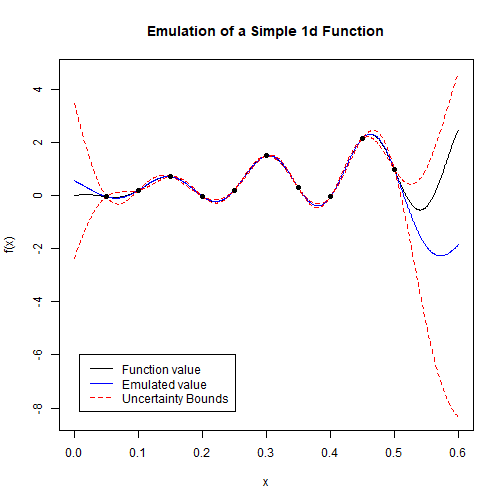plot of chunk plot-1d-first We can see a few things from this plot. The emulator does exactly replicate the function at the points used for training (the black dots in the plots), and the corresponding uncertainty is zero at these points. Away from these points, the emulator does a good job of interpolating the function values, represented by the coincidence of the black and blue lines: the exception to this is areas far from a training point (the edges of the plot). However, we can see that even where the emulator expectation diverges from the function value, the both lines lie inside the uncertainty bounds (here demonstrated by the red lines). ## History Matching We have a trained emulator, which we can see performs well over the region of interest. Suppose we want to find input points which result in a given output value. While with this function it would be straightforward to find such input points (either analytically or numerically), in general this would not be the case. We can therefore follow the history matching approach, using the emulator as a surrogate for the function. History Matching consists of the following steps: • Train emulators on known points in the target space; • Use the trained emulators to rule out regions of parameter space that definitely cannot give rise to the desired output value; • Sample a new set of points from the remaining space (the ‘non-implausible’ region); • Input these new points into the model/simulator/function to obtain a new training set. These four steps are repeated until either 1) we have a suitably large number of points producing the desired output; 2) the whole parameter space has been ruled out; 3) The emulators are as confident evaluating the parameter space of interest as the model itself. Here, we will not worry about these stopping conditions and instead perform the steps to complete two waves of emulation and history matching. The first thing we require is a target output value: suppose we want to find points $$x$$ such that $$f(x)=0$$, up to some uncertainty. We define this as follows: target1d <- list(f = list(val = 0, sigma = 0.05)) This means of defining targets, while common in real-life observations where we observe an outcome with some measurement error, may not be available to a particular output. An alternative is to define the target as a range of values that could be taken: for example in this case we could define a similar target to the above as target1d_alt <- list(f = c(-0.1, 0.1)) The generate_new_design function is used to propose new points. There are a multitude of different methods that can be used to propose the new points: in this particular one-dimensional case, it makes sense to take the most basic approach. This is to generate a large number of space-filling points, reject those that the emulator rules out as implausible, and select the subset of the remaining points that has the maximal minimum distance between them (so as to cover as much of the non-implausible space as possible). new_points1d <- generate_new_design(em1d, 10, target1d, method = 'lhs') #> Proposing from LHS... #> Enough points generated from LHD - no need to apply other methods. #> Selecting final points using maximin criterion... The necessary parameters here are the (list of) emulators, the number of points (here, 10), and the target(s). Having obtained these new points, we include them on our previous plot, along with the target bounds, to demonstrate the logic of the point proposal. We will also include a bar indicating the implausibility at each value of x: the implausibility is defined roughly as the distance between the emulator prediction and the target value, normalised by the uncertainty at that point (both the emulator uncertainty em$get_cov(x) and the observational uncertainty target1d$sigma). We will define the implausibility more rigorously shortly, but for now it signifies the level of ‘suitability’ of a point; lower values of implausibility equate to points deemed more suitable. col_func <- function(x, max_val) { if (x <= 3) return(rgb(221*x/3, 255, 0, maxColorValue = 256)) return(rgb(221+34*(x-3)/(max_val-3), 255*(1-(x-3)/(max_val-3)), 0, maxColorValue = 256)) } imps <- em1d$f$implausibility(plot1d$x, target1d$f) col.pal <- purrr::map_chr(imps, col_func, max(imps)) plot(data = plot1d, f ~ x, ylim = c(min(plot1d[,-1]-1), max(plot1d[,-1])), type = 'l', main = "Emulation of a Simple 1d Function", xlab = "x", ylab = "f(x)") lines(data = plot1d, E ~ x, col = 'blue') lines(data = plot1d, max ~ x, col = 'red', lty = 2) lines(data = plot1d, min ~ x, col = 'red', lty = 2) points(data = data1d, f ~ x, pch = 16, cex = 1) abline(h = target1d$f$val, lty = 2) abline(h = target1d$f$val + 3*target1d$f$sigma, lty = 2) abline(h = target1d$f$val - 3*target1d$f$sigma, lty = 2) points(unlist(new_points1d, use.names = F), y = func(unlist(new_points1d, use.names = F)), pch = 16, col = 'blue') points(plot1d$x, rep(min(plot1d[,-1])-0.5, length(plot1d$x)), col = col.pal, pch = 16) legend('bottomleft', inset = c(0.05, 0.1), legend = c("Function value", "Emulated value", "Uncertainty Bounds"), col = c('black', 'blue', 'red'), lty = c(1,1,2))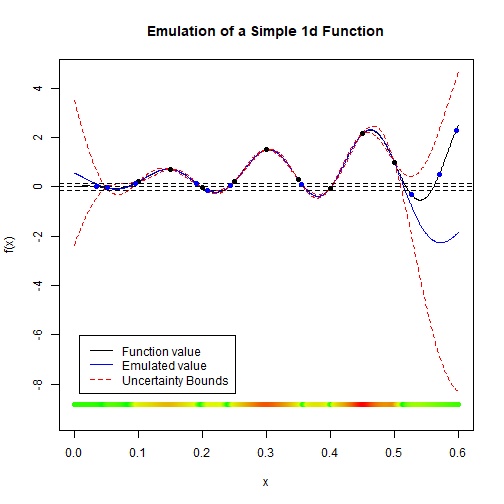plot of chunk plot-1d-results-first Here, the colour bar at the bottom indicates the implausibility: the more green the value at a point, the more acceptable the emulator thinks the point is for matching the target. Note that some of the newly proposed points (in blue) do not live inside the target bounds - in particular, there are points on the far right that are not near the target. In these regions, the implausibility is also quite green, even though we know by inspection that they will not match the target. This is a consequence of the way that the emulator proposes points: because the emulator uncertainty is large in that region of the parameter space, it cannot rule out those regions with certainty, and therefore samples from that region. This is in contrast to many optimisation methods, which look for regions that satisfy the conditions: the history matching iteratively removes regions that cannot satisfy the conditions. Because of the built-in understanding of uncertainty, the history matching is highly unlikely to remove parts of parameter space that could eventually result in an adequate match to the targets. The points in the high uncertainty region will be extremely instructive in training a second wave of emulators, which may consequently remove the space. ## Second Wave The second wave is very similar to the first, so little needs to be explained. new_data1d <- data.frame(x = unlist(new_points1d, use.names = F), f = func(unlist(new_points1d, use.names = F))) em1d_2 <- emulator_from_data(new_data1d, c('f'), ranges1d) #> Fitting regression surfaces... #> Building correlation structures... #> Creating emulators... #> Performing Bayes linear adjustment... plot1d_2 <- data.frame( x = plot1d$x, f = plot1d$f, E = em1d_2$f$get_exp(test1d), min = em1d_2$f$get_exp(test1d) - 3*sqrt(abs(em1d_2$f$get_cov(test1d))), max = em1d_2$f$get_exp(test1d) + 3*sqrt(abs(em1d_2$f$get_cov(test1d))) ) plot(data = plot1d_2, f ~ x, ylim = c(min(plot1d_2[,-1]), max(plot1d_2[,-1])), type = 'l', main = "Emulator of a Simple 1-dimensional Function: Wave 2", xlab = "Parameter value", ylab = "Function value") lines(data = plot1d_2, E ~ x, col = 'blue') lines(data = plot1d_2, max ~ x, col = 'red', lty = 2) lines(data = plot1d_2, min ~ x, col = 'red', lty = 2) points(data = new_data1d, f ~ x, pch = 16, cex = 1) legend('topleft', inset = c(0.05, 0.05), legend = c("Function value", "Emulated value", "Uncertainty Bounds"), col = c('black', 'blue', 'red'), lty = c(1,1,2))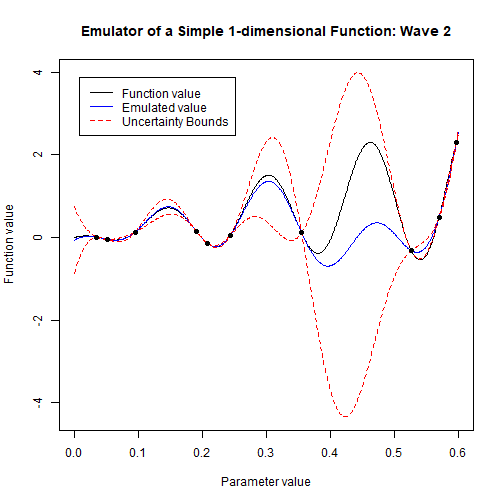plot of chunk wave-2-1d This plot underlines the importance of using all waves of emulation. The first wave was trained over most of the space, and so gives a moderately confident estimate of the function on the interval $$[0, 0.5]$$. The second wave emulator is trained only on regions that are of interest at this point, and so is less confident than the first wave emulator in parts of parameter space. However, we still have the emulator from the first wave, which contains all the information of the previous training runs by design. In this wave of history matching, therefore, we use both the first- and second-wave emulator to propose points. new_new_points1d <- generate_new_design(c(em1d_2, em1d), 10, z = target1d, method = 'lhs') #> Proposing from LHS... #> Enough points generated from LHD - no need to apply other methods. #> Selecting final points using maximin criterion... plot(data = plot1d_2, f ~ x, ylim = c(min(plot1d_2[,-1]), max(plot1d_2[,-1])), type = 'l', main = "Emulator of a Simple 1-dimensional Function: Wave 2", xlab = "Parameter value", ylab = "Function value") lines(data = plot1d_2, E ~ x, col = 'blue') lines(data = plot1d_2, max ~ x, col = 'red', lty = 2) lines(data = plot1d_2, min ~ x, col = 'red', lty = 2) points(data = new_data1d, f ~ x, pch = 16, cex = 1) legend('topleft', inset = c(0.05, 0.05), legend = c("Function value", "Emulated value (wave 2)", "Uncertainty Bounds"), col = c('black', 'blue', 'red'), lty = c(1,1,2)) abline(h = target1d$f$val, lty = 2) abline(h = target1d$f$val + 3*target1d$f$sigma, lty = 2) abline(h = target1d$f$val - 3*target1d$f$sigma, lty = 2) points(x = unlist(new_new_points1d, use.names = F), y = func(unlist(new_new_points1d, use.names = F)), pch = 16, col = 'blue')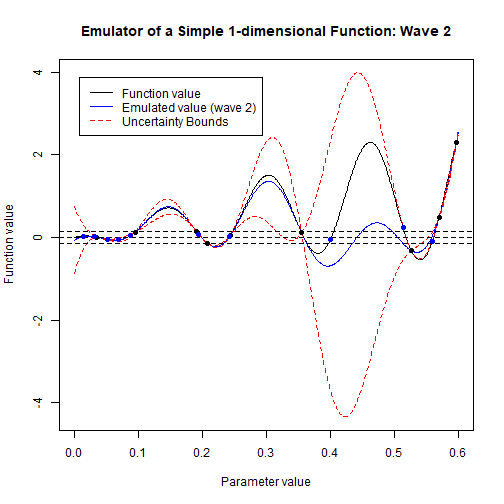plot of chunk gen-1d-points-2 We can see that the proposed points are much better overall than the first wave points. Because the second-wave emulator has much greater certainty in the region $$[0.5, 0.6]$$, it is far better at determining suitability of points in that region. From the plot, it looks like there should be points proposed from the central regions where the wave-two emulator is uncertain, but the fact that we are also using the first-wave emulator as a metric for point proposal means that these points are ruled out anyway. # Two-dimensional example We now consider a slightly higher-dimensional example, in order to consider emulator diagnostics and some more interesting plots. Consider the following pair of functions in two dimensions: $$f_1(x) = 2\cos(1.2x-2) + 3\sin(-0.8y+1)$$ $$f_2(x) = y\sin x - 3\cos(xy)$$ The associated parameter space to explore is given by $$x\in[-\pi/2, \pi/2]$$ and $$y\in[-1,1]$$. We create a set of initial data to train emulators on: here we select the initial points to be space-filling using a Latin Hypercube design (package lhs): func1 <- function(x) { 2*cos(1.2*x[]-2) + 3*sin(-0.8*x[]+1) } func2 <- function(x) { x[]*sin(x[]) - 3*cos(x[]*x[]) } initial_data <- setNames(data.frame(sweep(maximinLHS(20, 2) - 1/2, 2, c(pi, 2), "*")), c('x', 'y')) validation_data <- setNames(data.frame(sweep(maximinLHS(20, 2) - 1/2, 2, c(pi, 2), "*")), c('x', 'y')) initial_data$f1 <- apply(initial_data, 1, func1)
initial_data$f2 <- apply(initial_data, 1, func2) validation_data$f1 <- apply(validation_data, 1, func1)
validation_data$f2 <- apply(validation_data, 1, func2) In this example, we have created two data sets: a training set initial_data and a validation set validation_data. This will allow us to see the performance of the emulators on previously untested points. In the one-dimensional case, this was not necessary as we can quite easily visualise the performance of the emulator using the simple plots. As in the one-dimensional case, we will define a target to match to. In this case, we’ll match to the value given when $$x=0.1, y=0.4$$ - this means that for this example we know that the target can be matched to, and gives us a check that the emulators are not ruling out a region of parameter space that they shouldn’t be. ranges2d <- list(x = c(-pi/2, pi/2), y = c(-1, 1)) targets2d <- list( f1 = list(val = func1(c(0.1, 0.4)), sigma = sqrt(0.1)), f2 = list(val = func2(c(0.1, 0.4)), sigma = sqrt(0.005)) ) We have placed a much tighter bound of $$f_2$$, suggesting that this target should be harder to hit. We construct some emulators as in the one-dimensional case: ems2d <- emulator_from_data(initial_data, c('f1','f2'), ranges2d) #> Fitting regression surfaces... #> Building correlation structures... #> Creating emulators... #> Performing Bayes linear adjustment... ems2d #>$f1
#> Parameters and ranges:  x: c(-1.5708, 1.5708): y: c(-1, 1)
#> Specifications:
#>   Basis functions:  (Intercept); x; y; I(x^2); I(y^2)
#>   Active variables x; y
#>   Regression Surface Expectation:  1.7063; 2.3457; -1.064; 1.2272; -0.8021
#>   Regression surface Variance (eigenvalues):  0; 0; 0; 0; 0
#> Correlation Structure:
#> Bayes-adjusted emulator - prior specifications listed.
#>   Variance (Representative):  0.1616857
#>   Expectation:  0
#>   Correlation type: exp_sq
#>   Hyperparameters:  theta: 0.6433
#>   Nugget term: 0
#> Mixed covariance:  0 0 0 0 0
#>
#> $f2 #> Parameters and ranges: x: c(-1.5708, 1.5708): y: c(-1, 1) #> Specifications: #> Basis functions: (Intercept) #> Active variables x; y #> Regression Surface Expectation: -2.6381 #> Regression surface Variance (eigenvalues): 0 #> Correlation Structure: #> Bayes-adjusted emulator - prior specifications listed. #> Variance (Representative): 0.7324902 #> Expectation: 0 #> Correlation type: exp_sq #> Hyperparameters: theta: 1 #> Nugget term: 0.05 #> Mixed covariance: 0 We obtain a list of trained emulators. We note that both emulators have a dependence on the parameters, and their squares, which we would expect for this model. Since the emulators fit a quadratic surface to the data, and the data is generated by trigonometric functions, the closest global structure they can produce is quadratic. We want to check that the emulators are suitable for proposing new points robustly. We will check the following things: • We want the emulator prediction to ‘match’ the model prediction; by which we wouldn’t expect the emulator prediction to be frequently more than $$3\sigma$$ away from the model prediction in the regions that matter. • In general, we want the standardised errors of the emulator predictions relative to the model predictions to be not too large, but not too small. Large errors imply that the emulators are doing a bad job of fitting to validation points; consistently small errors imply that the emulators are underconfident and thus makes the wave of emulation less valuable for cutting out space. • We want to ensure that the emulators do not rule out any regions of space that the model would not. The converse is okay, and indeed expected (the emulators are going to be more conservative about ruling out points), but the emulators should absolutely not rule out regions of space that may be valid. These three tests are contained within validation_diagnostics, which prints out the corresponding plots for each emulator and returns any points which fail one or more of these checks. invalid_points <- validation_diagnostics(ems2d, targets2d, validation_data, plt = TRUE, row = 2)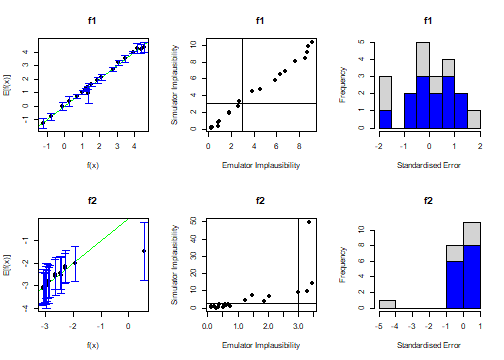plot of chunk validate-2d-ems Looking at these plots shows that the emulators are doing fine. Any failing points would be flagged as red in the first two columns of the plots, and appear in the data.frame invalid_points: should we have such points it is useful to consider why they might be problematic (for example, a point at an edge or a corner of the parameter space can have strange behaviours). At this stage, we can make a variety of changes to the prior specifications in order to combat these issues if they occur, by utilising the mult_sigma and set_hyperparams functions of the Emulator object. For instance, to inflate the emulator variance by a factor of 2 and decrease the correlation length to 0.5 for the $$f_1$$ emulator: modified_em <- ems2d$f1$mult_sigma(2)$set_hyperparams(list(theta = 0.5))
new_invalid <- validation_diagnostics(list(f1 = modified_em, f2 = ems2d$f2), targets2d, validation_data, plt = TRUE, row = 2)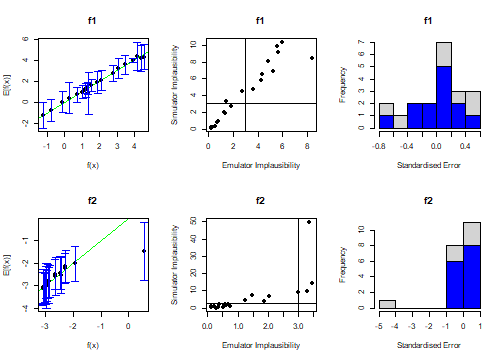plot of chunk changed-2d-validation The mult_sigma and set_hyperparams functions create a new Emulator object, allowing us to chain the two functions mult_sigma and set_hyperparams above, so in general we would replace the old emulator with the new one rather than combining lists (e.g. ems2d$f1 <- emd2d$f1$mult_sigma(...)). In this case, such tinkering is not required and hence we did not replace the $$f_1$$ emulator in situ. We can also use the set_sigma function to define the structure of the variance, including giving it functional dependence on the outputs; this is beyond the need or scope of this example, however.

## Emulator Plots

The hmer package allows us to create plots analogous to those made by-hand in the one-dimensional case. The corresponding plots are contour plots over the input region.

emulator_plot(ems2d)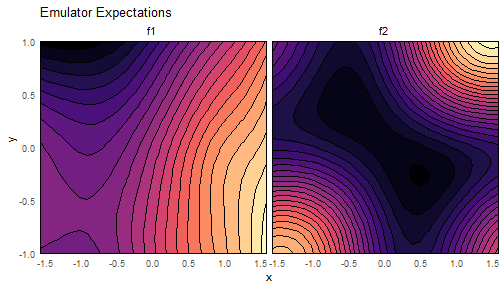plot of chunk plot-2d-ems

emulator_plot(ems2d, plot_type = 'sd')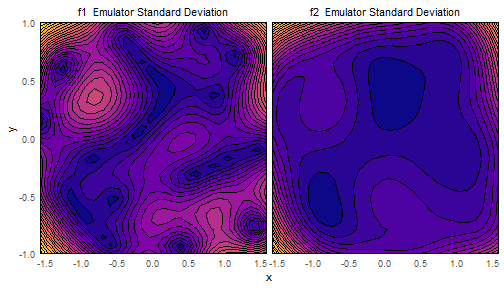plot of chunk plot-2d-ems

The emulator_plot function can produce a number of things: the default is an expectation plot equivalent to the blue line in the one dimensional case. The plot_type command determines what is plotted: ‘var’ has it plot the variance and ‘sd’ the standard deviation, which play the part of the distance from blue line to red dotted lines in the one-dimensional case. These combined plots are used mainly for diagnostics - to obtain an expectation or variance plot with meaningful scale, we plot them one-by-one. As they are all ggplot objects, we can augment them after the fact.

emulator_plot(ems2d$f1, plot_type = 'sd') + geom_point(data = initial_data, aes(x = x, y = y))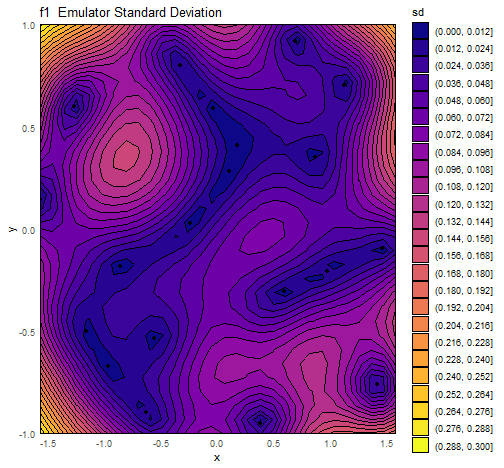plot of chunk plot-2d-ems-with-augment The structure of this plot warrants some discussion. In the one-dimensional case, we discussed the fact that the emulator was ‘certain’ at training points, and less so elsewhere; the uncertainty of the emulator was driven by its distance from a known training point. Here we have the same picture: minima of the emulator uncertainty (coloured here in deep blue) sit over training points. As we move away from a training point, the uncertainty increases until at the edges (which represent the maximum distance from training points) it reaches a maximum. The gradient of this change in uncertainty is controlled by the correlation length - a higher correlation length presumes that nearby points are more closely correlated, and in some sense can be equated to the ‘smoothness’ of our output space. We can modify the f1 emulator to have a larger correlation length and see what the effect is. inflated_corr <- ems2d$f1$set_hyperparams(list(theta = 1)) emulator_plot(inflated_corr, 'sd') + geom_point(data = initial_data, aes(x = x, y = y))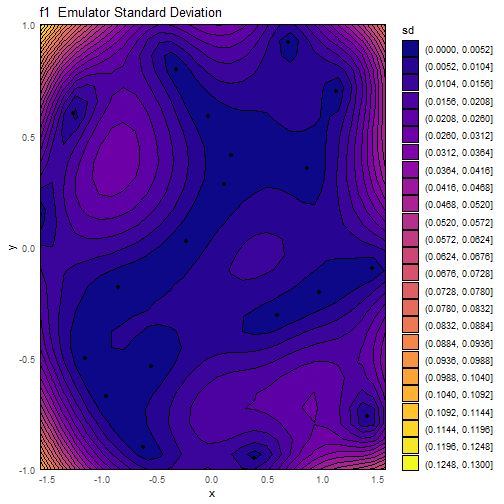plot of chunk plot-2d-ems-corr We can see that the emulator with the higher correlation length maintains a lower uncertainty when not at training points. The final two plots that emulator_plot can provide are those of implausibility, and nth-maximum implausibility. emulator_plot(ems2d, plot_type = 'imp', targets = targets2d)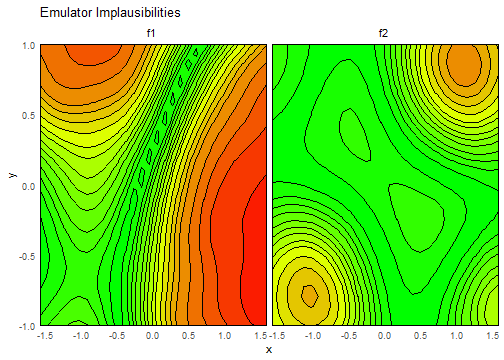plot of chunk plot-2d-ems-imp emulator_plot(ems2d, plot_type = 'nimp', targets = targets2d)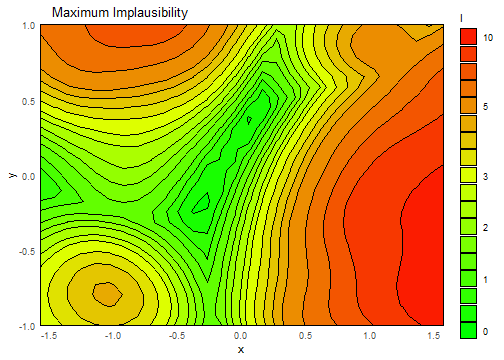plot of chunk plot-2d-ems-imp The implausibility of a point $$x$$, given a target $$z$$, is determined by the following expression: $$I(x)^2 = \frac{(\mathbb{E}[f(x)]-z)^2}{\text{Var}[f(x)] + \sigma^2}.$$ Here $$\mathbb{E}[f(x)]$$ is the emulator expectation at the point, $$\text{Var}[f(x)]$$ is the emulator variance, and $$\sigma^2$$ corresponds to all other sources of uncertainty outside of the emulators (e.g. observation error, model discrepancy, …). The smaller the implausibility at a given point, the more likely an emulator is to propose it as a new point. We can note that there are two reasons that implausibility might be small: either the numerator is small (in which case the difference between the emulator prediction and the target value is small, implying a ‘good’ point) or the denominator is large (in which case the point is in a region of parameter space that the emulator is uncertain about). Both are useful: the first for obvious reasons; the second because proposing points in such regions will make subsequent waves of emulators more certain about that region and thus allow us to reduce the allowed parameter space. The first plot here shows implausibility for each of the outputs. Of course, we want proposed points to be acceptable to matching both $$f_1$$ and $$f_2$$. The second plot gives maximum implausibility, which is exactly as it sounds: the largest implausibility across all outputs at a point. We can see that the $$f_1$$ emulator drives much of the space reduction in the lower-right and upper-left quadrant of the region, while the $$f_2$$ emulator drives space reduction in the lower-left and (to some extent) upper-right. This maximum implausibility is equivalent to the determination made by the one-dimensional emulator, where in that case the non-implausible region was easy to see graphically. We can now generate new points for a second wave of emulation. We again make a slight modification to the normal behaviour of the generate_new_design function. By default it will use a space-filling argument before using those points to draw lines to the boundaries of the non-implausible space - the points on the boundary are included in the proposal. From this augmented set, we perform importance sampling to try to fill out the space. To further ensure that the non-implausible space is filled out, we usually thin the proposed points and re-propose using the boundary search and importance sampling. This ‘resampling’ step can be repeated as many times as one desires (at a computational cost) and is useful in large-dimensional spaces; here we set resample = 0 for efficiency’s sake. new_points2d <- generate_new_design(ems2d, 40, targets2d, resample = 0) #> Proposing from LHS... #> LHS has high yield; no other methods required. #> Selecting final points using maximin criterion... new_data2d <- data.frame(x = new_points2d$x, y = new_points2d$y, f1 = apply(new_points2d, 1, func1), f2 = apply(new_points2d, 1, func2)) plot_wrap(new_data2d[,1:2], ranges2d)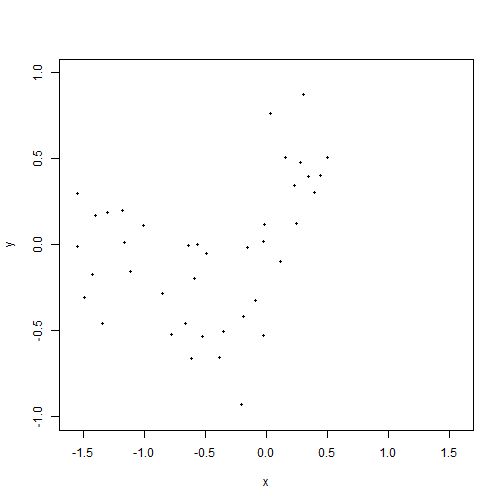plot of chunk gen-2d-points-first With these new points, we want to train a new set of emulators. We generated 40 points from generate_new_design so as to split them into a training set and a validation set once more: sample2d <- sample(40, 20) train2d <- new_data2d[sample2d,] valid2d <- new_data2d[-sample2d,] We train the new emulators, but first we redefine the range over which the emulators are defined. We can see from the plot above that any points with $$x>0.5$$ are not going to produce an acceptable match: it makes sense, therefore, to train the new emulators on a reduced part of parameter space. This reduction ensures that the emulators focus on interpolation between known points rather than extrapolation to a region we know is going to be unacceptable. This can be done within the emulator training by adding check.ranges = TRUE to the call to emulator_from_data; here we will just manually change the ranges with a small buffer. new_ranges2d <- list(x = c(-pi/2, 0.6), y = c(-1, 1)) ems2d_2 <- emulator_from_data(train2d, c('f1', 'f2'), new_ranges2d) #> Fitting regression surfaces... #> Building correlation structures... #> Creating emulators... #> Performing Bayes linear adjustment... ems2d_2 #>$f1
#> Parameters and ranges:  x: c(-1.5708, 0.6): y: c(-1, 1)
#> Specifications:
#>   Basis functions:  (Intercept); x; y; I(x^2); I(y^2)
#>   Active variables x; y
#>   Regression Surface Expectation:  0.8266; 1.2309; -1.316; 1.4056; -0.881
#>   Regression surface Variance (eigenvalues):  0; 0; 0; 0; 0
#> Correlation Structure:
#> Bayes-adjusted emulator - prior specifications listed.
#>   Variance (Representative):  0.04766416
#>   Expectation:  0
#>   Correlation type: exp_sq
#>   Hyperparameters:  theta: 1
#>   Nugget term: 0
#> Mixed covariance:  0 0 0 0 0
#>
#> $f2 #> Parameters and ranges: x: c(-1.5708, 0.6): y: c(-1, 1) #> Specifications: #> Basis functions: (Intercept) #> Active variables x; y #> Regression Surface Expectation: -2.8179 #> Regression surface Variance (eigenvalues): 0 #> Correlation Structure: #> Bayes-adjusted emulator - prior specifications listed. #> Variance (Representative): 0.1481509 #> Expectation: 0 #> Correlation type: exp_sq #> Hyperparameters: theta: 1 #> Nugget term: 0.05 #> Mixed covariance: 0 One thing we can see from this is that the emulators’ global variances have reduced, as one would expect. Since these emulators are trained on a smaller parameter space, and given more relevant points, the region of interest can be more accurately emulated. We check again on the emulator diagnostics and modify as necessary: invalid_points2 <- validation_diagnostics(ems2d_2, targets2d, valid2d, plt = TRUE, row = 2)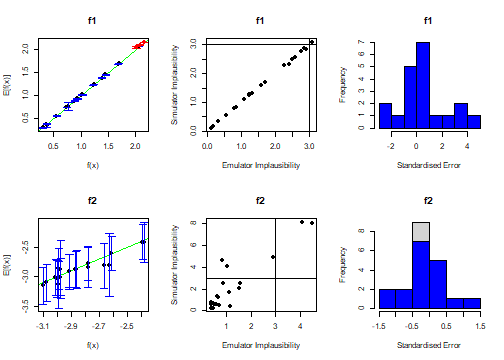plot of chunk validate-2d-ems-2 Again, we modify the emulators with reference to these plots. It appears that the emulator for output $$f_1$$ would benefit from some tweaking, which we do here. ems2d_2$f1 <- ems2d_2$f1$mult_sigma(1.4)$set_hyperparams(list(theta = 1/3)) We briefly look at the implausibilities for these new emulators: emulator_plot(ems2d_2, 'imp', targets = targets2d)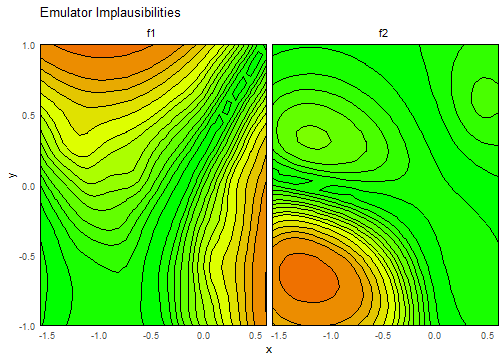plot of chunk plot-2d-imp-2 Finally, we propose points from a combination of this wave and the previous wave. new_new_points2d <- generate_new_design(c(ems2d_2, ems2d), 40, targets2d, resample = 0) #> Proposing from LHS... #> LHS has high yield; no other methods required. #> Selecting final points using maximin criterion... plot_wrap(new_new_points2d, ranges2d)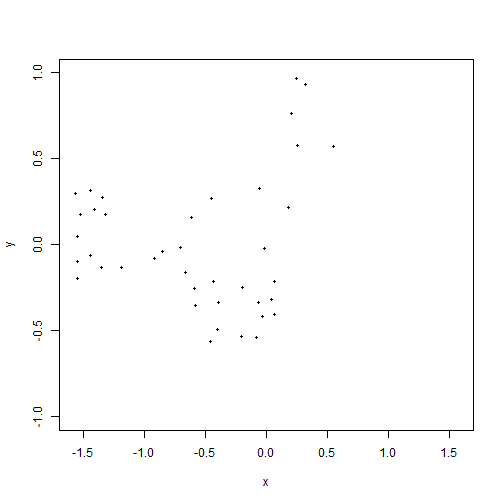plot of chunk gen-2d-points-2 For completeness, we record the function values of these new points: new_new_data2d <- data.frame(x = new_new_points2d$x, y = new_new_points2d$y, f1 = apply(new_new_points2d, 1, func1), f2 = apply(new_new_points2d, 1, func2)) We conclude this example by looking at the evolution of the allowed space, and the relative performance of the proposed points. A variety of functions are available to do so: we first package up all our waves into a list. all_waves <- list(rbind(initial_data, validation_data), new_data2d, new_new_data2d) We can view the output in three main ways: looking at the pure performance of the runs relative to the targets, looking at the distribution of the proposed points as the waves progress, or looking at the distribution of the output points as the waves progress. The functions simulator_plot, wave_points, and wave_values do just that. simulator_plot(all_waves, z = targets2d)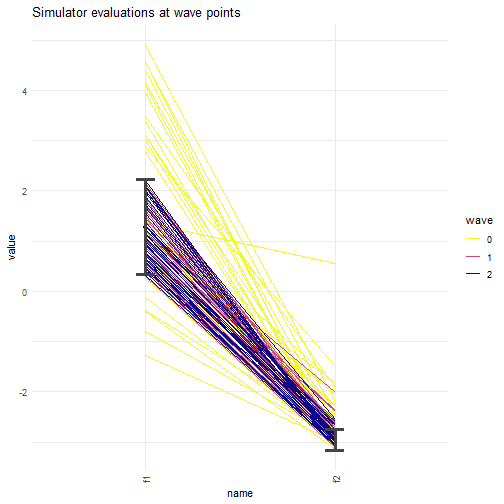plot of chunk plot-2d-waves wave_points(all_waves, names(ranges2d))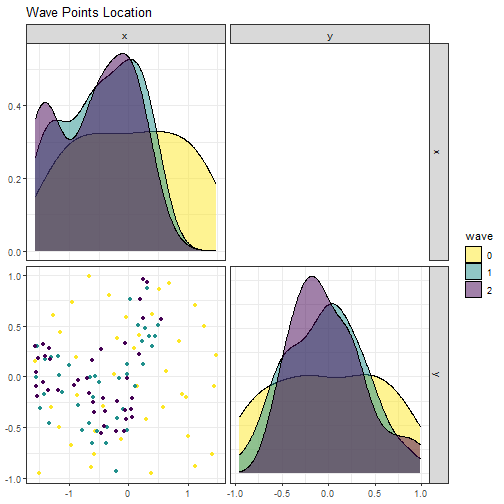plot of chunk plot-2d-waves wave_values(all_waves, targets2d, l_wid = 0.8)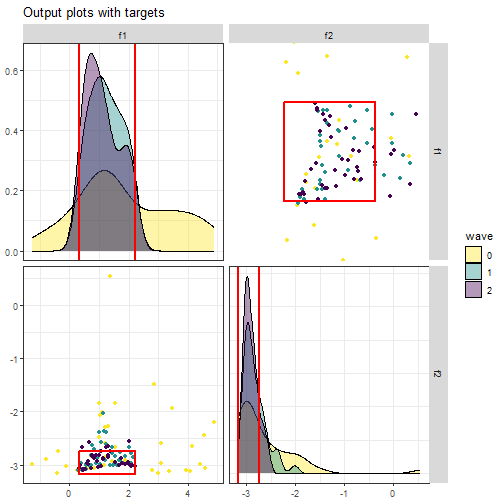plot of chunk plot-2d-waves We can see that, as the colour gets darker (representing later waves), the proposed points settle into the bounds of the targets. In fact, in this example, we can start to consider whether further waves are necessary - the points proposed at the end of the first wave were predominantly suitable for representing the non-implausible region. We can consider this by looking at the emulator uncertainty in the second wave compared to the observation uncertainty. c(ems2d_2$f1$u_sigma, ems2d_2$f2$u_sigma) #>  0.3056497 0.3849037 c(targets2d$f1$sigma, targets2d$f2\$sigma)
#>  0.31622777 0.07071068

The first emulator has uncertainty far lower than the target itself (about one quarter of the target sigma). Recall that the denominator of the implausibility measure combines all sources of uncertainty in quadrature: for $$f_1$$, therefore, the observation uncertainty will drive the behaviour of that uncertainty measure. Since we cannot reduce the observational error, subsequent waves are unlikely to have any large impact on the non-implausible space for $$f_1$$. For the second emulator, however, the emulator uncertainty is still relatively large, in comparison to the observation error, so we could perhaps consider training an additional wave only to the output of $$f_2$$. Alternatively, if we believe that the ‘yield’ of good points (i.e. those which hit all targets) is good enough at this wave, we could simply generate many more points at this wave and use those as a final representative sample of the non-implausible space. The decision on when we have performed enough waves is situational and dependent on one’s own intuition and needs; nevertheless, with two waves of emulation and history matching here we are in a strong position to be able to make that decision, supported by the information provided by the process.

### The Structure of a Bayes Linear Emulator

The basic structure of an emulator $$f(x)$$ is

$$f(x) = \sum_i \beta_i h_i(x) + u(x),$$

where the first term represents a regression surface (encapsulating the global behaviour of the function), and the second term accounts for local variations by defining an correlation structure on the space (more of which later). Our prior beliefs about the emulator must be specified. We need only second-order specifications (expectation and variance), so one can see that we must specify the following: - A set of basis functions, $$h(x)$$; - The second-order specifications for the coefficients $$\beta$$: namely the expectation and the variance $$\mathbb{E}[\beta]$$ and $$\text{Var}[\beta]$$;

• The second order specifications for the correlation structure: $$\mathbb{E}[u(x)]$$ and $$\text{Var}[u(x)]$$;

• The covariance between the coefficients and the correlation structure: $$\text{Cov}[\beta, u(x)]$$.

We could specify all of these things by hand; however, many of the parts of the above specification can be estimated quite readily automatically. The function emulator_from_data does exactly that, with a few assumptions. It assumes no covariance between the regression surface and the correlation structure, that the expectation of $$u(x)$$ is $$0$$, and that the variance of the coefficients $$\beta$$ is $$0$$ (i.e. that the regression surface is fixed and known); it also assumes that the correlation structure has an exponential-squared form. For two points $$x$$ and $$x^\prime$$, the correlation between them is

$$c(x,x^\prime) = \exp\left\{\frac{-(x-x^\prime)^2}{\theta^2}\right\}.$$

The closer two points are together, the higher their correlation; the parameter $$\theta$$ is known as a correlation length. The larger $$\theta$$, the larger the extent of the correlation between distant points. The emulator_from_data function also attempts to estimate the value of $$\theta$$.

The above analysis gives us a set of prior specifications for the emulator. However, it has not used all the information available from the training data. We can update our second-order beliefs with the Bayes Linear Update Equations - given data $$D$$ and prior specifications $$\mathbb{E}[f(x)]$$ and $$\text{Var}[f(x)]$$ for the emulator, the adjusted expectation and variance are
$$\mathbb{E}_D[f(x)] = \mathbb{E}[f(x)] + \text{Cov}[f(x), D]\text{Var}[D]^{-1}(D-\mathbb{E}[D]),$$ $$\text{Var}_D[f(x)] = \text{Var}[f(X)] - \text{Cov}[f(x), D]\text{Var}[D]^{-1}\text{Cov}[D, f(x)].$$